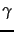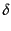Hey all! The class notes are not fully finished, but have come a long way with the addition of links to book chapters with our material. If the book chapters are too dense, I can rewrite them in my super less dense casual style – just give me a heads up.

Y’all know what to do!

At the Secret Convention of Logicians, the Master Logician placed a band on each attendee’s head, such that everyone else could see it but the person themselves could not. There were many, many different colors of band. The Logicians all sat in a circle, and the Master instructed them that a bell was to be rung in the forest at regular intervals: at the moment when a Logician knew the color on his own forehead, he was to leave at the next bell. Anyone who left at the wrong bell was clearly not a true Logician but an evil infiltrator and would be thrown out of the Convention post haste; but the Master reassures the group by stating that the puzzle would not be impossible for anybody present. How did they do it?

You have all been sent an email. Also, if you like your LaTeX editing in a Web 2.0 flavor, try out www.sharelatex.com

Today’s lab is meant to just be an extension of the day’s activities with most of the day left open for projects

Part 0: Variable Bit Encoding for Information Retrieval

Although I stressed it in class, it is worth saying again: IR is very concerned with space efficiency! So much so that significant work goes into the careful compression of postings lists. Consider, for example, the Reuter’s corpus, a commonly used and cited collection of around 800,000 documents. In general, each document in this corpus has around 200 tokens per document, 6 characters per token, and 100,000,000 postings. Uncompressed, this gives a corpus size of nearly 960MB and a postings list of 250MB.

Given a positional index

 encoding postings list the docIDs … 283042 283043 283044 283045 … gaps 1 1 1 … computer docIDs … 283047 283154 283159 283202 … gaps 107 5 43 … arachnocentric docIDs 252000 500100 gaps 252000 248100

we see that storing the gaps between the positions of term incidences in a document often means storing much smaller numbers. In these cases we can use fewer bits to encode the difference, but not in all cases. We need a variable bit encoding in order to store the odd large gap, here and there.

Variable Byte Encoding:

Recall that an integer is stored as four bytes. We will pretend that they are unsigned, or only taking on non-negative values. This means that we can use them to represent numbers from$0$ to$2^{32}-1$. However, we could certainly use fewer bits for numbers like 10 or 15 or even 511. A simple method for this is variable byte encoding. Given a number, we get its variable byte encoding by writing it first in binary, padding it at the front with 0s so that the number of bits used is a multiple of seven, then chunking the bits into groups of seven. The first group has a 1 appended to it to show that it is the first byte of the representation, then all other groups have a 0 appended to them. Take for example, 2056 is 100000001000 in straight binary. We chunk this into groups of at most seven as 10000 0001000 then pad it to 0010000 0001000. Finally we add one to the first chunk and zero to the rest to get 10010000 00001000, a two byte representation for 2056.

Write the following numbers in variable byte notation. Note that Google can convert numbers to binary for you!

1. 1
2. 1023
3. 12345678
What is the smallest number for which variable byte encoding is less efficient than standard four byte notation? In other words, for what number n does the variable byte representation of n require more bits than the standard four byte representation?
Variable Bit Codes:
The simplest variable bit code is unary coding. The number x is represented by x 1s followed by a single 0. This clearly not optimal! However, it does allow us to specify a number of bits to read. We can now represent each positive integer (and note that we don’t need zero if we are only representing gaps) as its binary encoding without a leading 1, preceded by the length of this representation in unary. This is called a gamma code.

For example, the number 15 is 1111 in binary, so it’s gamma code is 1110111. Woo, less than a whole byte for 15, just barely better than the above variable byte representation.

What is the following sequence of gaps?

111001011110000010111111010110

How many bits would we have needed to encode this with the standard 4 byte integer encoding?

A cool idea from the IR textbook.codes are relatively inefficient for large numbers  as they encode the length of the offset in inefficient unary code.codes differ fromcodes in that they encode the first part of the code (length) incode instead of unary code. The encoding of offset is the same. For example, thecode of 7 is 10,0,11 (again, we add commas for readability). 10,0 is thecode for length (2 in this case) and the encoding of offset (11) is unchanged. (i) Compute thecodes for the other numbers in Table 5.5 . For what range of numbers is thecode shorter than thecode? (ii)code beats variable byte code in Table 5.6 because the index contains stop words and thus many small gaps. Show that variable byte code is more compact if larger gaps dominate. (iii) Compare the compression ratios ofcode and variable byte code for a distribution of gaps dominated by large gaps.

Part 1: Final Projects

Part 2: Daily Feedback

An n-simplex is a collection of n+1 points which are connected by faces, edges, cells, etc. For example, a 1-simplex is a line segment, a 2-simplex is a triangle, and a 3-simplex is a tetrahedron. A regular simplex has all edges with equal length. Note that when we put a point on the center of every face of an n-simplex, we get a smaller n-simplex inside. For example, if we put a point in the middle of each edge of a triangle, then connect these points, we get a smaller triangle. If we put a point in the center of each face of a tetrahedron, then connect them, we get a smaller tetrahedron. Call this the inscribed simplex.

Given a regular 5-simplex, what is the ratio of the length of each edge connecting two points to the length of each edge in the inscribed simplex?

Today’s lab is all final projects! Keep in mind that your presentation will be a week from today, so if you need more resources, advice, etc, get in touch with me, and I will do what I can. Also, if you feel that your project is in a good place, you can always work on older projects, or find Ben or the RCs to learn something cool.